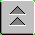Introduction to Naval Weapons Engineering

Introduction to SONAR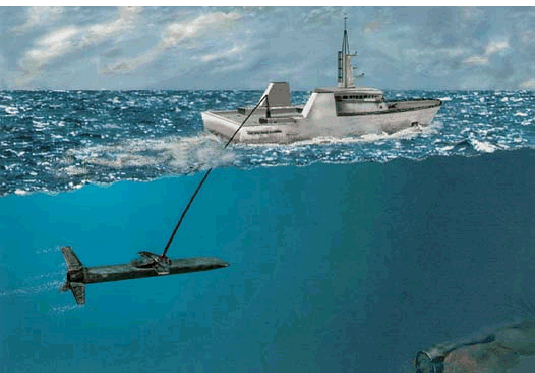SONAR (sound navigation and ranging) systems have many similarities to RADAR and electro-optical systems. Again, detection is based on the propagation of waves between the target and detector. There are active sonar systems, where the wave propagates from the transmitter to the target and back to the receiver, analogous to pulse-echo radar. There are also passive sonar systems, where the target is the source of the energy which propagates to the receiver, analogous to passive infrared detection. Therefore, a great deal of what has been discussed about these systems will also apply to sonar. Sonar, however, differs fundamentally from radar and electro-optics because the energy is transferred by acoustics waves propagating in water. So we first begin by discussing the characteristics of acoustic waves.

Acoustic Waves

The term acoustic refers to sound, either in water or air. A more precise terminology would be to label them as longitudinal waves. If we consider a string of objects, like weights, connected by springs, there will be two possible ways of propagating a disturbance down the string. The first method is to displace the weight in a direction perpendicular to the string. This will create a transverse wave. As the weight is moved off the axis, the springs from the adjacent weight will exert a restoring force that pulls it back on axis. The other method is to displace the weights along the axis of the string. This creates a longitudinal wave. Again the restoring force will tend to push the weight back into place.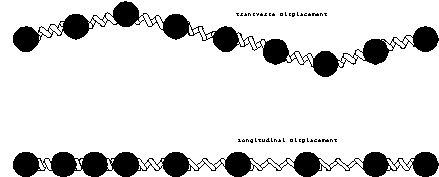Figure 1. Transverse and
Longitudinal Waves.

Water can be modeled as this string of weights connected by springs, but of course, it would be in three dimensions. The weights are the molecules of water, and the springs are the inter-molecular forces that tend to keep water together, but separated from each other. In other words, if you try to pull the water molecules apart from each other there will be a resistance. On the other hand if you try to push them closer together, there will also be resistance. So our model seems plausible. Therefore, we will call the longitudinal wave in water an acoustic wave. The disturbance of the medium which propagates is the distance between molecules. However this cannot be measured directly.

When water molecules are pushed or pulled apart, they exert a restoring force that resists the motion. Considering an extended body of water, the force will be felt locally as pressure, or force per unit area. So the fundamental parameter of an acoustic wave is pressure. The amplitude of the wave will be the peak pressure reached in one cycle.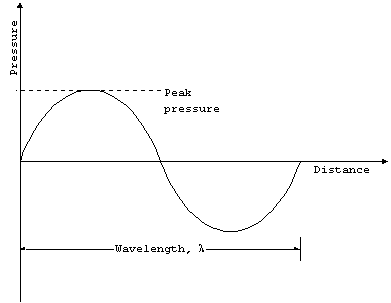Figure 2. Pressure Wave.

Propagation Speed

For a non-dispersive medium, which water is to some extent, we would also expect the same type of relationship between wavelength and frequency as with electromagnetic waves. The only difference is that the speed of propagation is slower, on the order of 1500 m/s in water, and it is no longer a constant. The speed of propagation depends on the ambient temperature, pressure and salinity of the water. Therefore we can write

fl = c(T,p,S)

where c(T,p,S) is the speed of propagation as a function of temperature (T), pressure (p) and salinity (S).

The speed of propagation is a fairly complicated function, and the effect of these three independent parameters are best stated as thumbrules:

1o C increase in temperature
3 m/s increase in speed.

100 meters of depth increase
1.7 m/s increase in speed.

1 ppt (part per thousand) increase in salinity
1.3 m/s increase in speed.

By far the greatest variation occurs in the ambient temperature. Changes by as much as 30o C are possible in the water in which a submarine operates. The equivalent change in depth which would change the propagation speed by the same amount is more than 5000 ft. Variations in salinity are limited to regions where fresh and salt water mix, which are normally beyond the regions where ASW operations take place.

Sound Pressure Level (SPL)

The fundamental parameters of an acoustic wave are the amplitude, which is a pressure, and the wavelength (or frequency). Pressure has units of force/area or in SI (system international) units, N/m2. The SI unit of pressure is the Pascal (Pa), where 1 Pa = 1 N/m2.

Unfortunately, most readers are not comfortable with this unit, so here is most of the commonly encountered units of pressure and their equivalents

Table 1. Common Units of Pressure.

 Units Normal atmospheric pressure atmospheres (atm) 1 bar 1.01325 Pascal (Pa) 1.01325 x 105 Pounds/in2 (psi) 14.6960 Inches Hg 29.9213 mm of Hg (torr) 760 Inches of Water 406.8 dynes/cm2 1.01325 x 106

Similarly to radar and electro-optics, we will need to describe the energy flow in an acoustic wave. The power per unit area in an acoustic wave varies as the square of the pressure. Apparently, in order to maximize confusion, the term intensity, I, refers to power per unit area, and therefore we write the proportionality:

I p2.

For working purposes, we can use a term called the sound pressure level, or SPL, which is defined as:

SPL 20 Log[p/(1 mPa)]

where 1 mPa is the reference value, similar to the way 1 mW is used in the definition of dBm. Therefore the exact coefficients of the proportionality are irrelevant, since SPL is a ratio. You should note the factor of 20 in front instead of the usual 10 used for decibels. Recall that decibels express a ratio of powers, which in this case are proportional to the square of pressure. Using the properties of logarithms, the exponent is brought down in front and multiplies the normal factor of 10. For example, 10 Log(x2) = 20 Log(x).

Acoustic analysis is done with logarithms in order to handle the wide range of values. Later when we write a maximum range equation, it will look totally different because everything will be expressed in decibels. As the first example, consider two acoustic waves. If they are of equal strength, they can be superimposed to create a signal with twice the intensity. In logarithms, this means the SPL will increase by +3 dB, since:

SPL1 = 20 Log(p1/1 mPa)= 10 Log {p12/(1 mPa)2}
SPL2 = 20 Log{p2/(1 mPa)}= 10 Log { p22/(1 mPa)2}

SPL2 = 10 Log{ 2p12/(1 mPa)2}
SPL2 = 10 Log{p12/(1 mPa)2} + 10 Log(2)

SPL2 = SPL1 + 10 Log(2), but 10 Log(2) = + 3,

SPL2 = SPL1 + 3

If you need to add together two source levels (SPL's), you cannot use ordinary addition, as the example shows. For instance, if SPL1 = SPL2 = 60 dB, you cannot combine the levels to get SPL 120 dB! The correct answer is 60 dB combined with 60 dB gives 63 dB. A shorthand notation that will remind us to apply special means to combine levels is the symbol "." For example, the correct equation should be

60 dB 60 dB = 63 dB.

Although tedious, we will now develop a systematic method for combining levels since we will need it shortly.

Combining Sound Pressure Levels

There are two common methods for combining sound pressure levels.

Calculator method (brute force). When two levels combine, you cannot simply add the peak pressures in the waves. You can only add the intensity which varies as pressure squared. To combine two levels, you must first "undo" the logarithm, add the pressure squared terms, then re-apply the logarithm. In detail:

SPL1 = 10 Log{ p12/(1 mPa)2}

p12/(1 mPa)2 = 10SPL1/10

SPL = 10 Log{ p12/(1 mPa)2 + p22/(1 mPa)2}

SPL = 10 Log{10SPL1/10 + 10SPL2/10}

Let's see if it works on the prototypical problem:

60 dB 60 dB = 10 Log{ 106 + 106} = 63 dB

Tabular method (easy way). When combining two sound pressure levels, you first find the difference between the levels, and then use the table to find the amount which is added to the larger of the two.

Table 2. Combining Decibels.

 Difference between sound pressure levels (SPL) Add this amount to larger: 0-1 3 2-4 2 5-9 1 >9 0

Again, to find 60 dB 60 dB, the difference between the two is zero add 3 to the larger:

60 dB 60 dB = 60 dB + 3 dB = 63 dB

Sources

Active Sonar

In an active sonar system, the source of the acoustic wave is part of the sonar system. The electrical energy from the transmitter must be converted into acoustic energy. The generic term for the device which accomplishes this task is transducer, which means something that converts the form of energy. If the transducer can only receive it is called a hydrophone, and if can only transmit it is called a projector. In many situations, the transducer can work both as transmitting and receiving device, which is the usual case for sonar, so it is just called a transducer. The output of the transducer is measured as an SPL, and is termed the source level (SL).

For a given total power output, the intensity will be reduced as a function of range because the same total power must be distributed over a larger area. Therefore, the SPL, which is the logarithm of intensity will drop with range from the source. We must measure SPL at a standard range from the source in order to be able to make meaningful comparisons. That standard range is one meter. Therefore SL is specified by an SPL measured at 1 m from the source. Sometimes, the notation will include a reference to the pressure unit and measurement distance. For example, SL = 145 dB//ref:1 mPa @ 1m, refers to a source level using a reference of 1 mPa measured at one meter. Here, we will omit this cumbersome notation since all source levels will specified using this reference.

Passive Sonar

In a passive sonar system, the source is the target itself. The same terminology applies to passive sources, namely the source level, SL. Of course, the source level is generally not known beforehand. However, source levels for hostile targets can be measured through intelligence gathering efforts, which cannot be discussed here. Passive sources fall into two main categories: broadband and narrowband sources.

Broadband sources, all the name suggest, create acoustic energy over a wide range of frequencies. This is similar to the thermal source in electro-optics. Typical broadband sources are noise from either the propeller/shaft, flow noise and some propulsion systems (example: steam). Noise from the propeller and shaft is generally at low frequency, meaning less than 1000 Hz. Furthermore, the rate of rotation can amplitude modulate the noise, which can be detected and demodulated to measure the shaft or propeller blade rate.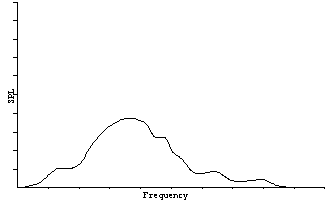Figure 3. Broadband source.

Narrowband sources radiate within a small band about a particular frequencies, or class of frequencies. Typical sources are the various pieces of machinery found in every ship. For example, pumps, motors, electrical generation equipment and propulsion systems. When specifying narrowband sources, it is important to also specify the frequency at which it occurs.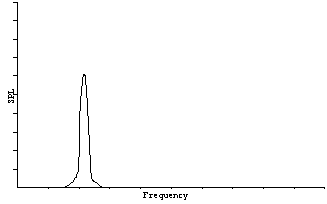Figure 4. Narrowband source.

Ambient Noise

The introduction of noise from other sources is a significant factor in sonar performance. There are a wide variety of noise sources present in the underwater environment, but the main, consistent contribution comes from only a few types. Ambient noise, unlike other sources, does not come from a particular direction or source. The noise level is the same everywhere in the local area. Therefore the SPL will be the same everywhere and it is not necessary to specify the range at which it was measured at (cf. Source level). So the ambient noise is specified as a particular SPL.

The most obvious contribution to the ambient noise is the action occurring on the surface of the ocean. The greater the size of the waves, the greater the ambient noise contribution. The waves are driven by the winds, so there is a direct correspondence between the steady wind speed and the sea state. The condition of the ocean surface is quantified by the sea state, which is a number ranging from 0-9. The Beaufort Wind Scale which ranges from 0-12 is the standard measure of the consistent wind speed.

Table 3. Beaufort Wind Scale and Sea States.

 Beaufort Wind speed (knots) Wave height (meters) Sea State 0 <1 0 0 1 1-3 2 4-6 0-0.1 1 3 7-10 0.1-0.5 2 4 11-16 0.5-1.25 3 5 17-21 1.25-2.5 4 6 22-27 2.5-4 5 7 28-33 4-6 6 8 34-40 9 41-47 10 48-55 6-9 7 11 56-63 9-14 8 12 >64 >14 9

The greater the wind speed or sea state, obviously the greater the ambient noise contribution. The frequency of the noise from sea state tends to be greater than 300 Hz.

The second main contribution to ambient noise comes from shipping in general. In regions where there are many transiting ships, the ambient noise will be increased substantially. This noise, in contrast to the noise from sea state, will be at low frequency (< 300 Hz). The amount of shipping traffic can be estimated by broad geographical considerations. For the purposes of estimating the ambient noise, we will arbitrarily describe locations as falling into one of six categories:

Table 4. Shipping Levels.

 Category Description Very remote No other ships present for many miles. Remote Infrequent distant ships. Quiet Occasional ship nearby. Shipping Lanes Many ships nearby. Heavy traffic (deep water) Constant passing of ships nearby, offshore. Heavy traffic (shallow water) Constant passing of ships nearby, inshore.

The two contributions to ambient noise, sea state and shipping can be predicted using the categories we have just defined. The noise as a function of frequency is found on a graph from a series of measurements called the Wenz curves.

Figure 5. Modified Wenz Curves.

To find the combined ambient noise level (AN), you must combine the sound pressure levels from the two curves using:

AN = Shipping (SPL) Sea State (SPL).

Example: predict the ambient noise at 300 Hz from shipping lanes and sea state 2.

The shipping noise is 65 dB
The sea state noise is 62 dB

65 dB 62 dB = 65 + 2 = 67 dB (tabular method)

AN = 67 dB

The third possible ambient noise source is biologics, meaning sea-life. These are as widely varied as they are unpredictable. One common source is snapping shrimp. Others include whales and dolphins. It is impossible to estimate the contribution from biologics to the ambient noise beforehand, therefore the effects are only known in-situ.

Self-Noise

There is another kind of noise in acoustic systems, that comes from the electronics itself, noise from the platform it is on, or from the flow of water across the receiving array itself. This is called self-noise, SN. Self-noise will have a minimum value which is present regardless of the speed that the array is traveling through the water, and then the self noise will increase due to flow noise and the increase in noise from the platform which carries it. Self noise is specified as a SPL, and has a frequency and speed dependence.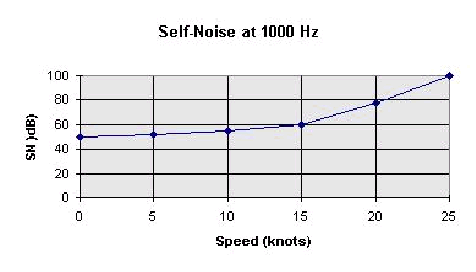Figure 6. Self-noise as a function of speed.

Total Noise

The ambient and self-noise must be combined, using the special method for combing sound pressure levels. We define the total noise level, NL as

NL AN SN

Detection of Acoustic Energy

Beam-forming

Transducers (or hydrophones) are used to receive acoustic energy. If they are designed to receive equally well in all directions, they are called omni-directional. The transducer can be constructed with some directionality in which case it will have some range of angles from which it can receive energy which will be its beamwidth, q. The beamwidth can only be made as small as the diffraction limit, meaning

q 2l/D

where l is the wavelength and D is the diameter of the aperture or face of the transducer. Transducers are usually small devices, on the order of 10 cm. However, they can be combined into arrays, similarly to the way simple antenna elements are used in phased array radar. Now the size of the array, or aperture, L, will determine the beamwidth.

q 2l/L.

When receiving, the narrow beamwidth will allow the array to reject more interfering noise, since the ambient noise comes from all directions. This is completely analogous to radar antenna gain, and we have a similar expression in sonar. It is a logarithmic term called the directivity index, DI. It is roughly the equivalent of antenna gain in decibels (i.e. 10 Log (G)). However, in sonar this term is applied only to the reception of energy, since the definition of SL accounts for the gain achieved in transmission.

As an example of directivity index, consider a linear array of hydrophones with overall length L. The array will form a beam perpendicular to the array with beamwidth, q 2l/L. The two-dimensional gain G = 2p/q pL/l. Therefore, the directivity index DI 10 Log (pL/l). The more precise value for this and the circular aperture of diameter D is given below. Note that the DI for the circular plate represents the three dimensional beam-forming whereas the linear array only forms a beam in a plane. The factor in front is 20 instead of 10 for this reason.

Table 5. Array directivities.

 Array/Shape Beamwidth, q DI Circular plate, diameter D (2.4)l/D 20 Log (pD/l) Linear array, length L 2l/L 10 Log (2L/l)

There are two limiting cases that apply to arrays of transducers:

Low frequency limit. In this case, the large wavelength causes a very large beamwidth and all directionality is lost. DI approaches 0. This occurs, for example when 2L = lmax, or

fmin = c/2L

fmax = c/d

High frequency limit. In this case, the wavelength becomes too short for effective steering of the beam. This condition occurs when the spacing between elements in the array is the same as the wavelength: d = l. This sets an upper limit on frequency,

Based on this analysis, the best overall frequency response would come from a sonar array that was very large with a large number of closely spaced transducers. Of course, this is also the most expensive, and will require the most processing capability for beam-forming.

Detection Criterion

Just like in the previous situations, the criterion for detection requires the amount of power which is collected by the receiver to exceed the noise level by some threshold. The ratio of signal-to-noise in logarithmic form is the SNR. The minimum SNR for detection is called the detection threshold, DT. Therefore detection generally occurs, meaning more than 50% of the time, whenever

SNR > DT.

We have already discussed the source of acoustic energy and the noise level, but have yet to describe the relationship between them. For now, describe the signal loss from source to receiver as transmission loss, TL. The transmission loss term includes all the effects of the energy spreading out, and attenuation, and various other effects which will be the main topic of discussion in the next two chapters. It is this term, in fact, which makes sonar much more difficult to predict and operate as compared to radar, for example.

So now the SNR at the receiver can be written explicitly for a passive system (which has a one-way transmission):

SNR = SL + DI - TL - NL

where:

SL = source level
TL = transmission loss
NL = noise level (AN SN)
DI = directivity index

For an active system, we have on additional term, which describes the reflection of energy from the target, called the target strength, TS. The target strength acts as a source level after reflection, and therefore includes any directional effects of reflection. The target strength is a function of the target size, surface material and shape in the same way that radar cross section varies. Also note for the active system, there is a two-way transmission loss, therefore

SNR = SL - TL + TS - TL - NL+ DI,

SNR = SL - 2TL + TS - NL + DI

Although these terms look similar in active and passive systems the values for each term will in general be quite different.

You should note that these equations use ordinary addition and subtraction even while working with sound pressure levels. This is absolutely correct. We are not combining sound pressure levels in these equations, we are actually multiplying and dividing terms. Recall that SNR is the logarithmic equivalent of the signal-to-noise ratio. When you take the logarithm, the division becomes subtraction. Likewise, terms which multiply, like the equivalent of gain, are now added. This is usually a source of great confusion: when to add/subtract and when to combine sound pressure levels using the special methods above. Here, we have been explicit in the use of the symbol "" whenever the special method is used.